# CBSEtips.in

## Wednesday 24 February 2021

### CBSE Class 8 Maths - MCQ and Online Tests - Unit 15 - Introduction to Graphs

#### CBSE Class 8 Maths – MCQ and Online Tests –  Unit 15 – Introduction to Graphs

Every year CBSE students attend Annual Assessment exams for 6,7,8,9,11th standards. These exams are very competitive to all the students. So our website provides online tests for all the 6,7,8,9,11th standards’ subjects. These tests are also very effective and useful for those who preparing for any competitive exams like Olympiad etc. It can boost their preparation level and confidence level by attempting these chapter wise online tests.

These online tests are based on latest CBSE syllabus. While attempting these, our students can identify their weak lessons and continuously practice those lessons for attaining high marks. It also helps to revise the NCERT textbooks thoroughly

#### CBSE Class 8 Maths – MCQ and Online Tests – Unit 15 – Introduction to Graphs

Observe the following bar graph and answer the related questions: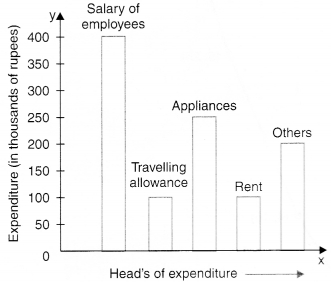Question 1.
What is the sum of the expenditures (in thousands of rupees) on travelling allowance and rent ?
(a) 100
(b) 200
(c) 300
(d) 400.

Hint:
100 + 100 = 200

Question 2.
(a) Travelling allowance/rent
(b) Appliances
(c) Salary of employees
(d) Others.

Hint:
The length of the bar corresponding to the head travelling allowance/rent is minimum.

Question 3.
On which two heads, is the expenditure same ?
(a) Salary of employees and others
(b) Travelling allowance and rent
(c) Appliances and rent
(d) Appliances and others.

Answer: (b) Travelling allowance and rent
Hint:
The lengths of the bars corresponding to the heads travelling allowance and rent are the same.

Question 4.
What is the difference of expenditures (in thousands of rupees) on salary of employees and rent ?
(a) 100
(b) 200
(c) 300
(d) 400.

Hint:
400 – 100 = 300

Question 5.
On which head, is the expenditure maximum ?
(a) Travelling allowance
(b) Rent
(c) Appliances
(d) Salary of employees.

Hint:
The length of the bar corresponding to the head ‘salary of employees’ is maximum.

Observe the following circle-graph and answer the related questions:Question 1.
What is the sum of the expenditures on food and education if the budget of the family is Rs 10800 ?
(a) Rs 5000
(b) Rs 8000
(c) Rs 5400
(d) Rs 6000.

Hint:
150 + 30 = 180
$$\frac{180}{360}$$ × 10800 = 5400

Question 2.
On which head is the expenditure minimum ?
(a) Education
(b) House rent
(c) Food
(d) Clothes.

Hint:
The central angle is minimum for education.

Question 3.
If the budget of the family is Rs 10800, what is the saving ?
(a) Rs 1050
(b) Rs 1000
(c) Rs 950
(d) Rs 1200.

Hint:
Saving = $$\frac{35}{360}$$ × 10800 = 1050.

Question 4.
On which head is the expenditure maximum ?
(a) Food
(b) Clothes
(c) House rent
(d) Education.

Hint:
The central angle is maximum for food.

Question 5.
What is the difference of expenditures on clothes and education if the budget of the family is Rs 10800 ?
(a) Rs 1200
(b) Rs 1000
(c) Rs 800
(d) Rs 1500.

Hint:
70 – 30 = 40
$$\frac{40}{360}$$ × 10800 = 1200.

Observe the following histogram and answer the related questions: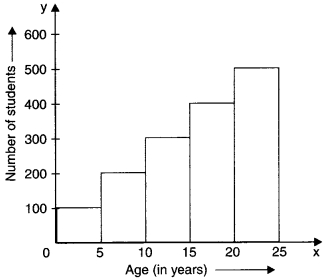Question 1.
The sum of the number of students in the class intervals 10-15 and 20-25 is
(a) 800
(b) 900
(c) 600
(d) 400.

Hint:
10-15 ? 300
20-25 ? 500
300 + 500 = 800.

Question 2.
In which class interval, are the minimum number of students ?
(a) 0-5
(b) 5-10
(c) 10-15
(d) 15-20.

Hint:
The length of the rectangle on 0 – 5 is maximum.

Question 3.
In which class intervals, is the number of students 200 ?
(a) 5-10
(b) 0-5
(c) 20-25
(d) 15-20.

Hint:
5-10 ? 200

Question 4.
In which class interval, are the maximum number of students ?
(a) 0-5
(b) 5-10
(c) 20-25
(d) 15-20.

Hint:
The length of the rectangle on 20 – 25 is maximum.

Question 5.
The difference in the number of students of class intervals 0-5 and 5-10 is
(a) 100
(b) 200
(c) 300
(d) 400.

Hint:
0-5 ? 100
5-10 ? 200
200-100 = 100

Observe the following temperature time graph and answer the related questions:Question 1.
What is the fall in temperature from 13 hours to 21 hours ?
(a) 2°F
(b) 3°F
(c) 4°F
(d) 6°F

Hint:
104 – 98 = 6

Question 2.
At what time(s) is the temperature minimum ?
(a) 7 hours and 21 hours
(b) 9 hours
(c) 11 hours
(d) 13 hours.

Answer: (a) 7 hours and 21 hours
Hint:
7, 21 ? 98°F

Question 3.
103°F temperature is at time
(a) 11 hours
(b) 13 hours
(c) 15 hours
(d) 21 hours.

Hint:
103°F ? 11 hours

Question 4.
What is the difference of temperatures at 7 hours and 21 hours ?
(a) 0°F
(b) 1°F
(c) 2°F
(d) 3°F.

Hint:
98-98 = 0

Question 5.
What is the rise in temperature from 11 hours to 13 hours ?
(a) 1°F
(b) 2°F
(c) 4°F
(d) 3°F.

Hint:
104 – 103 = 1

Question 6.
The coordinates of the origin are
(a ) (0, 0)
(b) (1, 0)
(c) (0, 1)
(d) (1, 1).

Hint:
0 ? (0, 0)

Question 7.
What are the coordinates of a point whose x-coordinate is 3 and y-coordinate is 4?
(a) (3, 3)
(b) (3, 4)
(c) (4, 3)
(d) (4, 3).

Question 8.
What are the coordinates of a point whose x-coordinate is 1 and y-coordinate isO?
(a) (1, 0)
(b) (0, 0)
(c) (0, 1)
(d) (1, 1).

Question 9.
What are the coordinates of a point whose x-coordinate is 0 and y-coordinate is 1?
(a) (0, 1)
(b) (0, 0)
(c) (1, o)
(d) (1, 1).

Observe the following velocity-time graph and answer the related questions: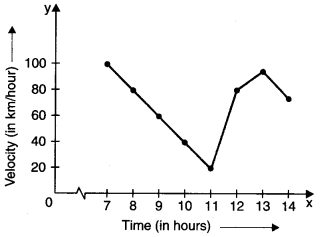Question 1.
At what time is the velocity minimum ?
(a) 8
(b) 9
(c) 10
(d) 11.

Hint:
11 ? 20

Question 2.
At what times are the velocities equal ?
(a) 8 and 12
(b) 9 and 11
(c) 7 and 12
(d) 11 and 13.

Hint:
8 ? 80
12 ? 80

Question 3.
What is the fall in velocity from 7 to 11 ?
(a) 80 km/hour
(b) 90 km/hour
(c) 100 km/hour
(d) 20 km/hour.

Hint:
100 – 20 = 80

Question 4.
What is the rise in velocity from 11 to 12?
(a) 10 km/hour
(b) 20 km/hour
(c) 30 km/hour
(d) 60 km/hour.

Hint:
80 – 20 = 60

Question 5.
At what time is the velocity maximum ?
(a) 7
(b) 8
(c) 9
(d) 10.

Hint:
7 ? 100

Observe the following runs-over graph and answer the related questions: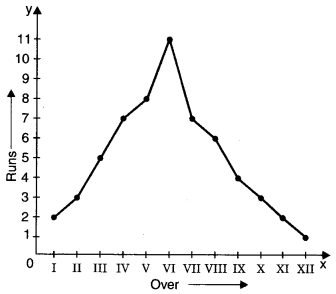Question 1.
3 runs are scored in which overs ?
(a) II and X
(b) I and V
(c) VII and VIII
(d) X and XII

Hint:
II ? 3, X ? 3

Question 2.
In which over are the minimum runs scored ?
(a) X
(b) XI
(c) XII
(d) IX.

Hint:
XII ? 1

Question 3.
What is the difference of runs scored in IV and V overs ?
(a) 1
(b) 2
(c) 3
(d) 4.

Hint:
8 – 7 = 1

Question 4.
What is the sum of runs scored in I and XII overs ?
(a) 1
(b) 2
(c) 3
(d) 4.

Hint:
2 + 1 = 3

Question 5.
In which over are the maximum runs scored ?
(a) II
(b) IV
(c) V
(d) VI.

Hint:
VI ? 11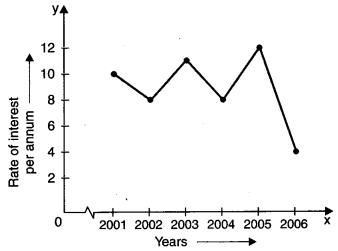Question 1.
The fall in interest from 2001 to 2002 was
(a) 1%
(b) 2%
(c) 3%
(d) 4%.

Hint:
10 – 8 = 2

Question 2.
In which year was the rate of interest minimum ?
(a) 2006
(b) 2004
(c) 2001
(d) 2005.

Hint:
2006 ? 4

Question 3.
The difference in the maximum and minimum rates of interest was
(a) 2%
(b) 4%
(c) 6%
(d) 8%.

Hint:
12 – 4 = 8

Question 4.
Rise in interest from 2004 to 2005 was
(a) 2%
(b) 4%
(c) 6%
(d) 8%.

Hint:
12 – 8 = 4

Question 5.
In which year was the rate of interest maximum ?
(a) 2005
(b) 2003
(c) 2006
(d) 2002.

Hint:
2005 ? 12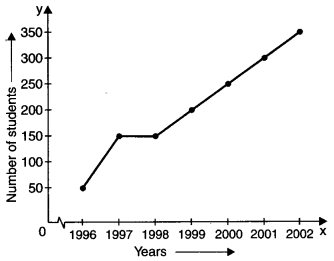Question 1.
In which year did 50 students appear ?
(a) 1998
(b) 2001
(c) 1997
(d) 1996.

Hint:
50 ? 1996

Question 2.
In which two years was the number of students appearing was the same ?
(a) 1997 and 1998
(b) 1998 and 1999
(c) 1999 and 2000
(d) 2000 and 2001.

Hint:
1997 ? 150
1998 ? 150

Question 3.
In which year did the maximum number of students appear ?
(a) 2002
(b) 2000
(c) 2001
(d) 1999.

Hint:
2002 ? 350

Question 4.
What is the maximum number of students that appeared in any year ?
(a) 350
(b) 300
(c) 250
(d) 300.

Hint:
350

Question 5.
How many students appeared in the year 2000 ?
(a) 200
(b) 250
(c) 300
(d) 350.

Hint:
2000 ? 250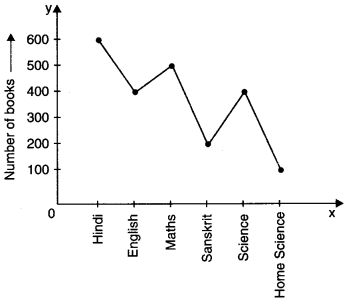Question 1.
How many books are there in Sanskrit and Home Science taken together ?
(a) 100
(b) 200
(c) 300
(d) 400.

Hint:
Sanskrit ? 200
Home Science ? 100
200 + 100 = 300

Question 2.
The minimum books are of which subject ?
(a) Home Science
(b) Sanskrit
(c) Science
(d) English

Hint:
Home Science ? 100

Question 3.
500 books are of which subject ?
(a) English
(b) Science
(c) Hindi
(d) Maths.

Hint:
500 ? Maths

Question 4.
The maximum books are of which subject ?
(a) Hindi
(b) Science
(c) English
(d) Sanskrit.

Hint:
Hindi ? 600

Question 5.
How many books are of subject Home Science ?
(a) 100
(b) 200
(c) 300
(d) 400.

Hint:
Home Science ? 100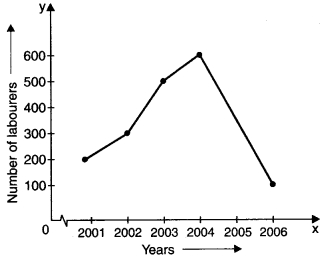Question 1.
Find the rise in the number of labourers from 2001 to 2004.
(a) 200
(b) 300
(c) 400
(d) 500.

Hint:
2001 ? 200
2004 ? 600
600 – 200 = 400

Question 2.
In which year was the number of labourers minimum ?
(a) 2003
(b) 2004
(c) 2005
(d) 2006.

Hint:
2006 ? 100

Question 3.
What was the difference of the number of labourers in the years 2002 and 2003 ?
(a) 100
(b) 200
(c) 300
(d) 400.

Hint:
2002 ? 300
2003 ? 500
500 – 300 = 200

Question 4.
In which year was the number of labourers maximum ?
(a) 2001
(b) 2002
(c) 2003
(d) 2004.

Hint:
2004 ? 500

Question 5.
Find the sum of the number of labourers in the years 2004 and 2006.
(a) 700
(b) 600
(c) 200
(d) 500.

Hint:
2004 ? 600
2006 ? 100
600 + 100 = 700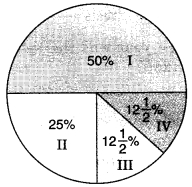Question 1.
A ________ is a bar graph that shows data in intervals.
(a) Bar-graph
(b) Pie-chart
(c) Histograph
(d) Line Graph

Question 2.
The number of students of class II is
(a) 500
(b) 250
(c) 125
(d) 100.

Hint:
$$\frac{25}{100}$$ x 1000 = 250

Question 3.
In which two classes is the number of students the same ?
(a) I and II
(b) I and III
(c) III and IV
(d) I and IV.

Hint:
III ? 12$$\frac{1}{2}$$%
IV ? 12$$\frac{1}{2}$$%

Question 4.
There are in all 1000 students in a school. The number of students of class I are
(a) 500
(b) 250
(c) 125
(d) none of these.

Hint:
$$\frac{50}{100}$$ × 1000 = 500

Question 5.
The minimum number of students in any class is
(a) 125
(b) 250
(c) 500
(d) 1000.

Hint:
$$\frac{25}{2}$$ × $$\frac{1000}{100}$$ = 125

Question 6.
The sum of the number of students of class III and class IV is
(a) 500
(b) 1000
(c) 50
(d) 250.

Hint:
125 + 125 = 250

Question 7.
A graph that displays data that changes continuously over periods of time is called:
(a) Bar-graph
(b) Pie-chart
(c) Histograph
(d) Line Graph

Question 8.
A line graph which is a whole unbroken line is called a:
(a) Linear graph
(b) Pie-chart
(c) Histograph
(d) Bar-graph

Question 9.
Which point lies of y-axis?
(a) (-2, 0)
(b) (2, 0)
(c) (0, -2)
(d) (2, -2)

Question 10.
The point (0, 0) lies at:
(a) x-axis
(b) y-axis
(c) origin
(d) None of the above

Question 11.
The point (4, 0) lies on which of the following?
(a) x-axis
(b) y-axis
(c) origin
(d) None of the above

Question 12.
The point (-2,-2) is:
(a) near to x-axis
(b) near to y-axis
(c) near to origin
(d) Equidistant from x-axis and y-axis.

Answer: (d) Equidistant from x-axis and y-axis.

Question 13.
The point (-2, 5) is nearer to:
(a) x-axis
(b) y-axis
(c) origin
(d) None of the above

Question 14.
The point (-5, 2) is nearer to:
(a) x-axis
(b) y-axis
(c) origin
(d) None of the above

Question 15.
If we join (-3,2), (-3,-3) and (-3,4), then we obtain:
(a) A triangle
(b) Straight-line without passing through origin
(c) Straight-line passing through origin
(d) None of the above

Answer: (b) Straight-line without passing through origin

Share: